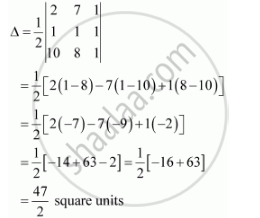# Find Area of the Triangle with Vertices at the Point Given in Each of the Following: (2, 7), (1, 1), (10, 8) - Mathematics

Find area of the triangle with vertices at the point given in each of the following:

(2, 7), (1, 1), (10, 8)

#### Solution

The area of the triangle with vertices (2, 7), (1, 1), (10, 8) is given by the relation,Is there an error in this question or solution?
Chapter 4: Determinants - Exercise 4.3 [Page 122]

#### APPEARS IN

NCERT Class 12 Maths
Chapter 4 Determinants
Exercise 4.3 | Q 1.2 | Page 122
Share